2020-05-27 09:45:43

## 学会这些 Python 美图技巧，就等着女朋友夸你吧

25 / 0 / 0 / 0

#### 一、前言

Python中有许多用于图像处理的库，像是Pillow，或者是OpenCV。而很多时候感觉学完了这些图像处理模块没有什么用，其实只是你不知道怎么用罢了。今天就给大家带了一些美图技巧，让你的图美翻全场，朋友圈赞不绝口，女朋友也夸你，富贵你好厉害啊！

#### 二、模块安装

pip install opencv-python
pip install pillow
pip install -i https://pypi.tuna.tsinghua.edu.cn/simple/ myqr  

#### 1、祛痘

import cv2
level = 22    # 降噪等级
img = cv2.bilateralFilter(img, level, level*2, level/2)    # 美颜
cv2.imwrite('result.jpg', img)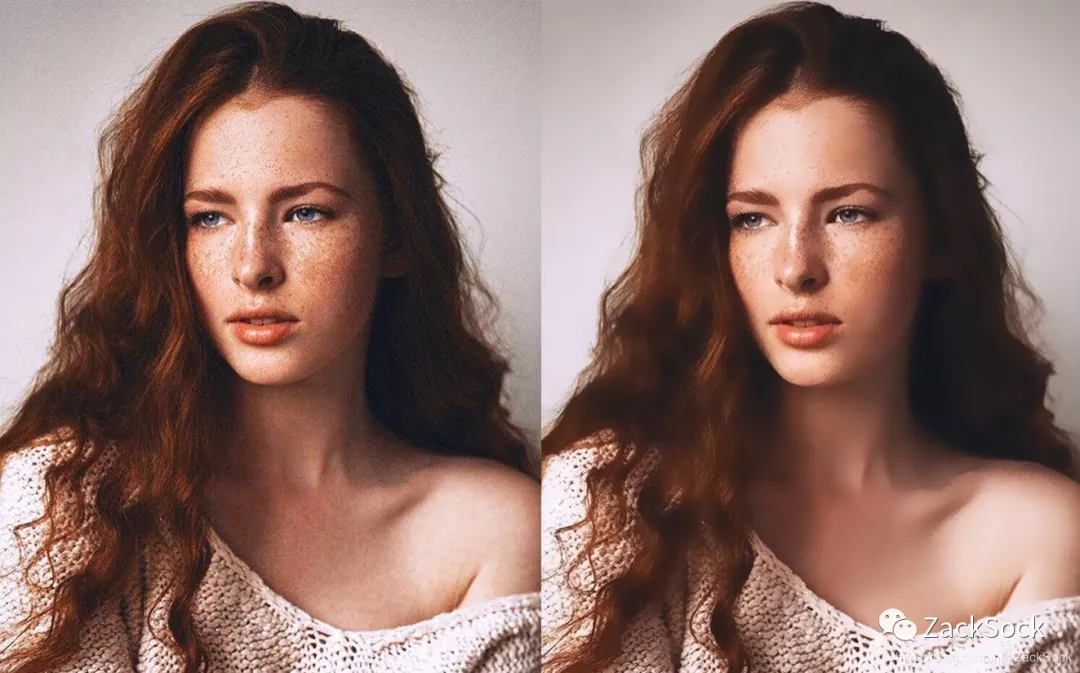#### 2、词云——我不只是一张图

from PIL import Image
import numpy as np
from wordcloud import WordCloud, ImageColorGenerator

# 读取背景图片

# 定义词云对象
wc = WordCloud(
# 设置词云背景为白色
background_color='white',
# 设置词云最大的字体
max_font_size=30,
# 设置词云轮廓
# 字体路径，如果需要生成中文词云，需要设置该属性，设置的字体需要支持中文
#font_path='msyh.ttc'
)
# 读取文本
# 生成词云
wc.generate(text)
# 给词云上色
# 保存词云
wc.to_file('result.png')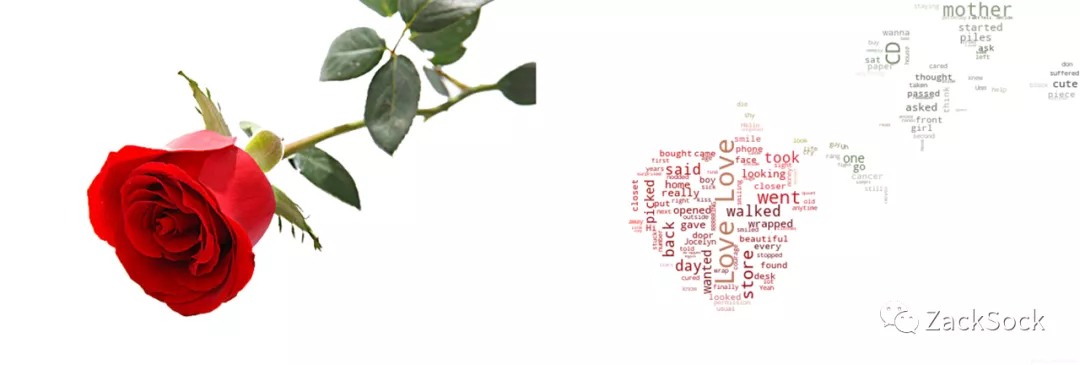#### 3、风格迁移——努力变成你喜欢的样子

import cv2
# 加载模型库
stylepro_artistic = hub.Module(name="stylepro_artistic")
# 进行风格迁移
im = stylepro_artistic.style_transfer(
images=[{
# 原图
# 风格图
}],
# 透明度
alpha = 0.1
)
# 从返回的数据中获取图片的ndarray对象
im = im['data']
# 保存结果图片
cv2.imwrite('result.jpg', im)#### 4、图中图——每一个像素都是你

import os
import cv2
import numpy as np

def getDominant(im):
"""获取主色调"""
b = int(round(np.mean(im[:, :, 0])))
g = int(round(np.mean(im[:, :, 1])))
r = int(round(np.mean(im[:, :, 2])))
return (b, g, r)

def getColors(path):
"""获取图片列表的色调表"""
colors = []

filelist = [path + i for i in os.listdir(path)]
for file in filelist:
im = cv2.imdecode(np.fromfile(file, dtype=np.uint8), -1)
dominant = getDominant(im)
colors.append(dominant)
return colors

def fitColor(color1, color2):
"""返回两个颜色之间的差异大小"""
b = color1 - color2
g = color1 - color2
r = color1 - color2
return abs(b) + abs(g) + abs(r)

def generate(im_path, imgs_path, box_size, multiple=1):
"""生成图片"""

# 读取图片列表
img_list = [imgs_path + i for i in os.listdir(imgs_path)]

# 读取图片
im = cv2.resize(im, (im.shape*multiple, im.shape*multiple))

# 获取图片宽高
width, height = im.shape, im.shape

# 遍历图片像素
for i in range(height // box_size+1):
for j in range(width // box_size+1):

# 图块起点坐标
start_x, start_y = j * box_size, i * box_size

# 初始化图片块的宽高
box_w, box_h = box_size, box_size

box_im = im[start_y:, start_x:]
if i == height // box_size:
box_h = box_im.shape
if j == width // box_size:
box_w = box_im.shape

if box_h == 0 or box_w == 0:
continue

# 获取主色调
dominant = getDominant(im[start_y:start_y+box_h, start_x:start_x+box_w])

img_loc = 0
# 差异，同主色调最大差异为255*3
dif = 255 * 3

# 遍历色调表，查找差异最小的图片
for index in range(colors.__len__()):
if fitColor(dominant, colors[index]) < dif:
dif = fitColor(dominant, colors[index])
img_loc = index

# 读取差异最小的图片
box_im = cv2.imdecode(np.fromfile(img_list[img_loc], dtype=np.uint8), -1)

# 转换成合适的大小
box_im = cv2.resize(box_im, (box_w, box_h))

# 铺垫色块
im[start_y:start_y+box_h, start_x:start_x+box_w] = box_im

j += box_w
i += box_h

return im

if __name__ == '__main__':

# 获取色调列表
colors = getColors('表情包/')
result_im = generate('main.jpg', '表情包/', 50, multiple=5)
cv2.imwrite('C:/Users/zaxwz/Desktop/result.jpg', result_im)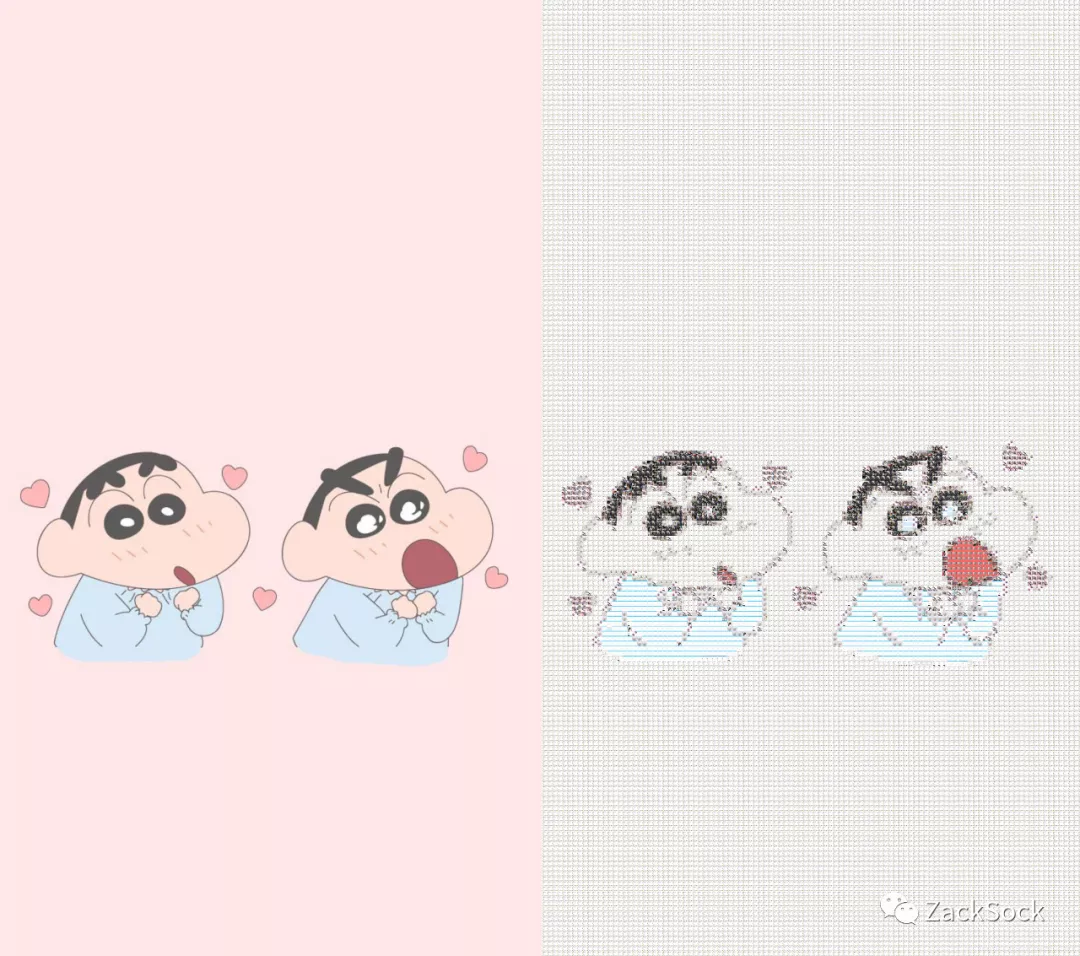#### 5、切换背景——带你去旅行

from PIL import Image
# 加载模型
humanseg = hub.Module(name='deeplabv3p_xception65_humanseg')
# 抠图
results = humanseg.segmentation(data={'image':['xscn.jpeg']})
# 读取背景图片
bg = Image.open('bg.jpg')
# 读取原图
im = Image.open('humanseg_output/xscn.png').convert('RGBA')
im.thumbnail((bg.size, bg.size))
# 分离通道
r, g, b, a = im.split()
# 将抠好的图片粘贴到背景上
bg.save('xscn.jpg')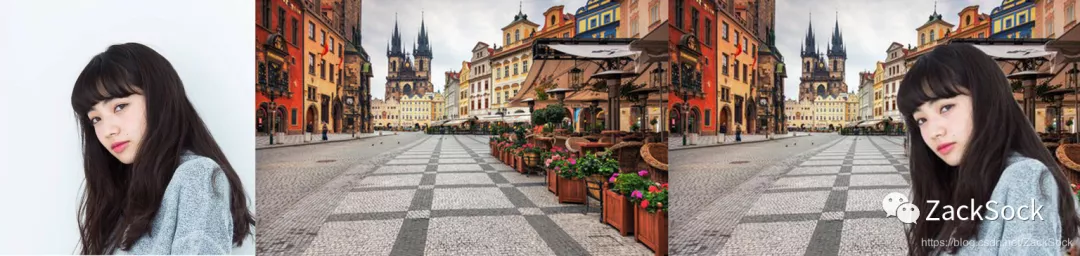#### 6、九宫格——一张照片装不下你的美

from PIL import Image
# 读取图片
im = Image.open('xscn.jpeg')
# 宽高各除 3，获取裁剪后的单张图片大小
width = im.size//3
height = im.size//3
# 裁剪图片的左上角坐标
start_x = 0
start_y = 0
# 用于给图片命名
im_name = 1
# 循环裁剪图片
for i in range(3):
for j in range(3):
# 裁剪图片并保存
crop = im.crop((start_x, start_y, start_x+width, start_y+height))
crop.save(str(im_name) + '.jpg')
# 将左上角坐标的 x 轴向右移动
start_x += width
im_name += 1
# 当第一行裁剪完后 x 继续从 0 开始裁剪
start_x = 0
# 裁剪第二行
start_y += height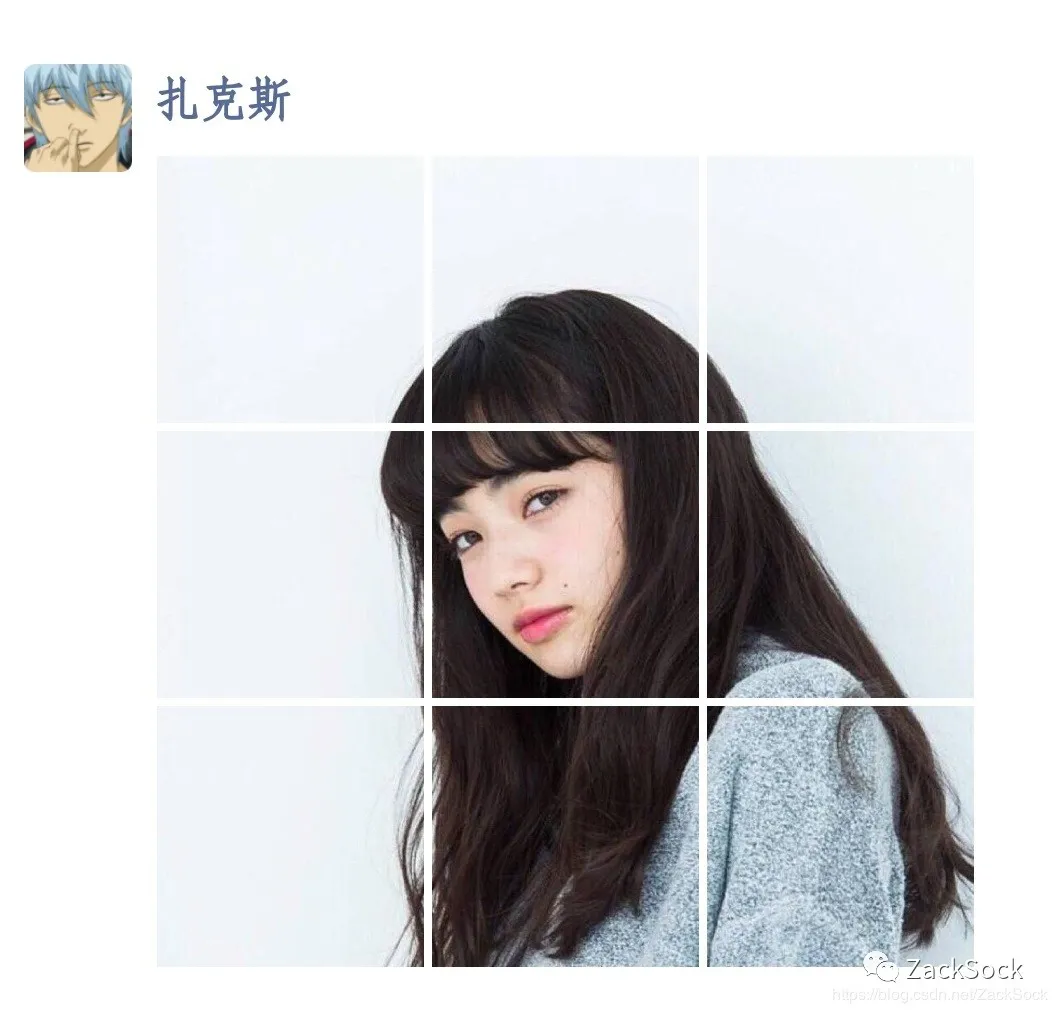在这里插入图片描述

#### 7、图片二维码——冰冷的图里也饱含深情

from MyQR import myqr
myqr.run(
words='http://www.baidu.com',    # 包含信息
picture='lbxx.jpg',            # 背景图片
colorized=True,            # 是否有颜色，如果为False则为黑白
save_name='code.png'    # 输出文件名
)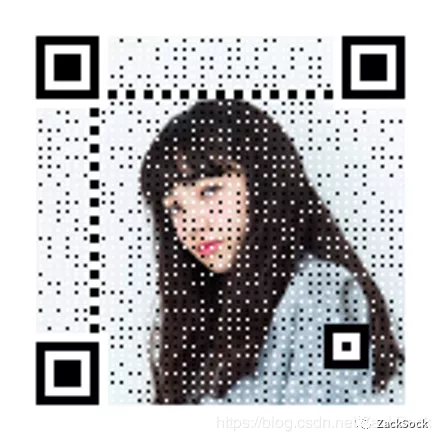在这里插入图片描述

PS: 如本文对您有疑惑，可加QQ：1752338621 进行讨论。

0
0